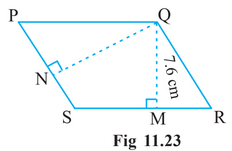# Q.  5.     is a parallelogram (Fig 11.23). is the height from to and is the height from to . If and              Find:(b) , if .

R Riya

We know that

Area of parallelogram

Here,

Base of parallelogram = 12 cm

and

Height of parallelogram = 7.6 cm

Now,

Area is also given by

Therefore, value of QN is

Exams
Articles
Questions